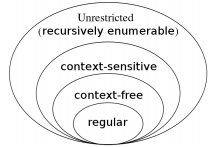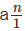Courses

# Pumping Lemma for Context Free Languages (CFLs) Computer Science Engineering (CSE) Notes | EduRev

## Computer Science Engineering (CSE) : Pumping Lemma for Context Free Languages (CFLs) Computer Science Engineering (CSE) Notes | EduRev

The document Pumping Lemma for Context Free Languages (CFLs) Computer Science Engineering (CSE) Notes | EduRev is a part of the Computer Science Engineering (CSE) Course Theory of Computation.
All you need of Computer Science Engineering (CSE) at this link: Computer Science Engineering (CSE)

Part V. Pumping Lemma for Context Free Languages (CFLs)
Consider the following figure:From the diagram, we can say that not all languages are context free languages. All context free languages are context sensitive and unrestricted. But all context sensitive and unrestricted languages are not context free from the above diagram.
We have a mechanism to show that some languages are not context free. The pumping lemma for CFLs allow us to show that some languages are not context free.

12 Pumping lemma for CFLs
Let G be a CFG. Then there exists a constant, n such that if W is in L(G) and |W| ≥ n, then we may write W = uvxyz such that,
1. |vy| ≥ 1 that is either v or y is non-empty,
2. |vxy| ≤ n then for all i ≥ 0,
uvixyiz is in L(G).
This is known as pumping lemma for context free languages.

Pumping lemma for CFLs can be used to prove that a language, L is not context free.
We assume L is context free. Then we apply pumping lemma to get a contradiction.
Following are the steps:
Step 1:
Assume L is context free. Let n be the natural number obtained by using the pumping lemma.
Step 2:
Choose W ∈ L so that |W| ≥ n. Write W = uvxyz using the pumping lemma.
Step 3:
Find a suitable k so that uvkxykz /∈ L. This is a contradiction, and so L is not context free.

Examples:
Example 1:
Show that L = {anbncn|n ≥ 1} is not context free.

Step 1:
Assume L is context free. Let n be the natural number obtained by using the pumping lemma.
Step 2:
Choose W ∈ L so that |W| ≥ n. Write W = uvxyz using the pumping lemma.
Let W = anbncn. Then |W| = 3n > n.

Write W = uvxyz, where |vy| ≥ 1, that is, at least one of v or x is not ε.
Step 3:
Find a suitable k so that uvkxykz /∈ L. This is a contradiction, and so L is not context free.
uvxyz = anbncn. As 1 ≤ |vy| ≤ n, v or x cannot contain all the three symbols a, b, c.

i) So v or y is of the form aibj(or bjcj) for some i,j such that i + j ≤ n. or
ii) v or y is a string formed by the repetition of only one symbol among a, b, c.
When v or y is of the form aibj, v2 = aibjaibj(or y2 = aibjaibj). As vis a substring of the form uv2xy2z,we cannot have uv2xy2z of the form ambmcm. So uv2xy2z ∉ L.

When both v and y are formed by the repetition of a single symbol (example: u = ai and v = bfor some iand j, i ≤ n, j ≤ n), the string uxz will contain the remaining symbol, say a1.Alsowill be a substring of uxz as a1 doesnot occur in v or y. The number of occurrences of one of the other two symbols in uxz is less than n (recall uvxyz = anbncn),and n is the number of occurrences of a1. So uv0xy0z = uxz ∉ L. Thus for any choice of v or y , we get a contradiction. Therefore, L is not context free.

Example 2:
Show that L = {ap|p is a prime number} is not a context free language.

This means, if w ∈ L, number of characters in w is a prime number.
Step 1:
Assume L is context free. Let n be the natural number obtained by using the pumping lemma.
Step 2:
Choose W ∈ L so that |W| ≥ n. Write W = uvxyz using the pumping lemma.
Let p be a prime number greater than n. Then w = ap ∈ L.

We write W = uvxyz
Step 3:
Find a suitable k so that uvkxykz /∈ L. This is a contradiction, and so L is not context free.
By pumping lemma, uv0xy0z = uxz ∈ L.
So |uxz| is a prime number, say q.
Let |vy| = r.
Then |uvqxyqz| = q + qr.
Since, q + qr is not prime, uvqxyqz ∉ L. This is a contradiction. Therefore, L is not context free.

Example 3:
Show that the language L = {anbncn|n ≥ 0} is not context free.

Step 1:
Assume L is context free. Let n be the natural number obtained by using the pumping lemma.
Step 2:
Choose W ∈ L so that |W| ≥ n. Write W = uvxyz using the pumping lemma.
Let W = anbncn.

We write W = uvxyz where |vy| ≥ 1 and |vxy| ≤ n, then
pumping lemma says that uvixyiz ∈ L for all i ≥ 0.

Observations:
a. But this is not possible; because if v or y contains two symbols from {a,b,c} then uv2xy2z contains a ’b’ before an ’a’or a ’c’ before ’b’, then uv2xy2z will not be in L.
b. In other case, if v and y each contains only a’s, b’s or only c’s, then uvixyi cannot contain equal number of a’s, b’s and c’s. So uvixyiz will not be in L.
c. If both v and y contains all three symbols a, b and c, then uv2xy2z will not be in L by the same logic as in observation (a).
So it is a contradiction to our statement. So L is not context free.

Step 3:
Find a suitable k so that uvkxykz ∉ L. This is a contradiction, and so L is not context free.

By pumping lemma, uv0xy0z = uxz ∈ L.
So |uxz| is a prime number, say q.
Let |vy| = r.
Then |uvqxyqz| = q + qr.
Since, q + qr is not prime, uvqxyqz ∉ L. This is a contradiction. Therefore, L is not context free.

Offer running on EduRev: Apply code STAYHOME200 to get INR 200 off on our premium plan EduRev Infinity!

## Theory of Computation

18 videos|43 docs|39 tests

,

,

,

,

,

,

,

,

,

,

,

,

,

,

,

,

,

,

,

,

,

;